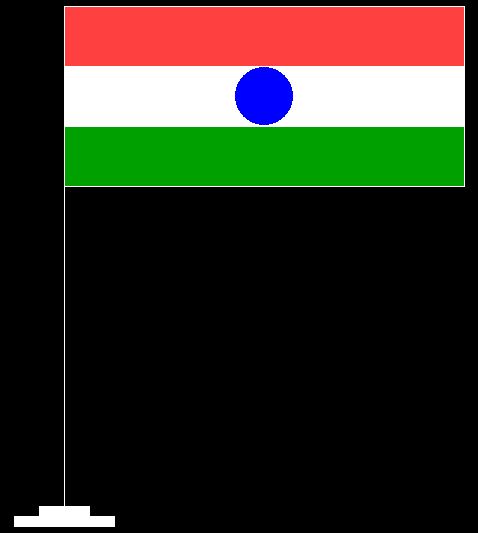Skip to content
Related Articles
C program to create Indian National Flag using Graphics
• Last Updated : 01 Apr, 2021

In this article, we will discuss how to draw the Indian National Flag using Graphics.

Approach:

• Draw a rectangle using the function rectangle().
• Equally, divide the above rectangle into three parts by creating the line using the function line().
• Draw double lines on each other i.e., draw 4 lines and among them, 2 lines will act as a divider between Red (Light red as there is no direct saffron color present in the graphics library) & White, and the other 2 lines will divide White and Green color.
• The Ashoka Chakra will be made using the function circle().
• To finish, all the spaces will be filled using the functions setfillstyle() and floodfill().

Below is the implementation of the above approach:

## C

 `// C program for the above approach ` ` `  `#include ` `#include ` `#include ` ` `  `// Driver Code ` `void` `main() ` `{ ` `    ``// Initialize of gdriver with ` `    ``// DETECT macros ` `    ``initgraph(&gd, &gm, ``"C:\\turboc3\\bgi"``); ` ` `  `    ``// Creating the base rectangle ` `    ``line(250, 100, 250, 600); ` `    ``line(250, 100, 250, 600); ` ` `  `    ``// Fil the White Color ` `    ``setfillstyle(SOLID_FILL, WHITE); ` ` `  `    ``// Create and fill the top strip ` `    ``rectangle(225, 600, 275, 610); ` `    ``rectangle(200, 610, 300, 620); ` ` `  `    ``floodfill(227, 608, 15); ` `    ``floodfill(202, 618, 15); ` ` `  `    ``// Fill the Light Red Color ` `    ``setfillstyle(SOLID_FILL, LIGHTRED); ` ` `  `    ``// Create and fill the ashoka ` `    ``// chakra with Blue ` `    ``rectangle(250, 100, 650, 280); ` `    ``line(250, 160, 650, 160); ` `    ``floodfill(252, 158, 15); ` ` `  `    ``// Fill the Blue Color ` `    ``setfillstyle(SOLID_FILL, BLUE); ` ` `  `    ``// Create and fill the left ` `    ``// part of the middle strip ` ` `  `    ``// Create a Circle ` `    ``circle(450, 190, 30); ` `    ``floodfill(452, 188, 15); ` ` `  `    ``// Fill the White Color ` `    ``setfillstyle(SOLID_FILL, WHITE); ` ` `  `    ``// Create and fill the right ` `    ``// part of the middle strip ` `    ``line(250, 160, 480, 160); ` `    ``line(250, 220, 480, 220); ` `    ``floodfill(252, 162, 15); ` ` `  `    ``// Fill the White Color ` `    ``setfillstyle(SOLID_FILL, WHITE); ` ` `  `    ``// Create and fill the bottom ` `    ``// strip ` `    ``line(480, 160, 650, 160); ` `    ``line(480, 220, 650, 220); ` `    ``floodfill(482, 162, 15); ` ` `  `    ``// Fill the Green Color ` `    ``setfillstyle(SOLID_FILL, GREEN); ` ` `  `    ``line(250, 220, 650, 220); ` `    ``floodfill(252, 278, 15); ` ` `  `    ``// Close the initialized gdriver ` `    ``closegraph(); ` `} `

Output:Want to learn from the best curated videos and practice problems, check out the C Foundation Course for Basic to Advanced C.

My Personal Notes arrow_drop_up
Recommended Articles
Page :